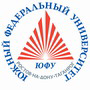Russian©  M. E. Abramyan (Southern Federal University, Shenzhen MSU-BIT University), 1998–2023

 Main Tasks Examples PT for MPI-2

 Overview Input-output operations Task groups Begin Integer Boolean If Case For While Series Proc Func Minmax Array Matrix String File Text Param Recur Dynamic Dynamic (obj) Tree Tree (obj)

# Conditional statement

Tasks If1–If3 have been slightly modified in version 4.15.

If1. An integer is given. If the integer is positive then decrease it by 8, otherwise do not change it. Output the obtained integer.

If2. An integer is given. If the integer is positive then decrease it by 8, otherwise increase it by 6. Output the obtained integer.

If3. An integer is given. If the integer is positive then decrease it by 8, if the integer is negative then increase it by 6, if the integer equals 0 then change it to 10. Output the obtained integer.

If4°. Three integers are given. Find the amount of positive integers in the input data.

If5. Three integers are given. Find the amount of positive and amount of negative integers in the input data.

If6°. Given two real numbers, output the larger value of them.

If7. Given two real numbers, output the order number of the smaller of them.

If8°. Given two real numbers, output the larger value and then the smaller value of them.

If9. The values of two real variables A and B are given. Redistribute the values so that A and B have the smaller and the larger value respectively. Output the new values of the variables A and B.

If10. The values of two integer variables A and B are given. If the values are not equal then assign the sum of given values to each variable, otherwise assign zero value to each variable. Output the new values of the variables A and B.

If11. The values of two integer variables A and B are given. If the values are not equal then assign the larger value to each variable, otherwise assign zero value to each variable. Output the new values of the variables A and B.

If12°. Given three real numbers, output the minimal value of them.

If13. Given three real numbers, output the value between the minimum and the maximum.

If14. Given three real numbers, output the minimal value and then the maximal value.

If15. Given three real numbers, output the sum of two largest values.

If16. The values of three real variables A, BC are given. If the values are in ascending order then double them, otherwise replace the value of each variable by its opposite value. Output the new values of the variables A, BC.

If17. The values of three real variables A, BC are given. If the values are in ascending or descending order then double them, otherwise replace the value of each variable by its opposite value. Output the new values of the variables A, BC.

If18. Three integers are given. One of them differs from two other equal integers. Output the order number of the integer that differs from the others.

If19. Four integers are given. One of them differs from three other equal integers. Output the order number of the integer that differs from the others.

If20. Three points A, BC on the real axis are given. Determine whether B or C is closer to A. Output the nearest point and its distance from A.

If21. Integer coordinates of a point in the coordinate plane are given. If the point coincides with the origin of coordinates then output 0, otherwise if the point lies on the x-axis or y-axis then output 1 or 2 respectively. If the point does not lie on the coordinate axes then output 3.

If22°. Given coordinates of a point that does not lie on the coordinate axes, find the number of a coordinate quarter containing the point.

If23. Given integer coordinates of three vertices of a rectangle whose sides are parallel to coordinate axes, find the coordinates of the fourth vertex of the rectangle.

If24. Given a real independent variable x, find the value of a real function f defined as:

 f(x) = 2·sin(x), if x > 0, 6 − x, if x ≤ 0.

If25. Given an integer independent variable x, find the value of an integer function f defined as:

 f(x) = 2·x, if x < −2 or x > 2, −3·x otherwise.

If26°. Given a real independent variable x, find the value of a real function f defined as:

 −x, if x ≤ 0, f(x) = x2, if 0 < x < 2, 4, if x ≥ 2.

If27. Given a real independent variable x, find the value of an integer function f defined as:

 0, if x < 0, f(x) = 1, if x belongs to [0, 1), [2, 3), …, −1, if x belongs to [1, 2), [3, 4), … .

If28. Given a number of year (as a positive integer), find the amount of days in the year. Note that the length of year is 365 days for an ordinary year and 366 days for a leap year. A leap year is every year whose number is divisible by 4, as 1980, except centenary years that are not divisible by 400 (for example, 1300 and 1900 are ordinary years, 1200 and 2000 are leap years).

If29. Given an integer, output its description string as: "negative even number", "zero number", "positive odd number", etc.

If30. An integer in the range 1 to 999 is given. Output its description string as: "two-digit even number", "three-digit odd number", etc.Designed byM. E. Abramyan and V. N. Braguilevsky Last revised:01.01.2023